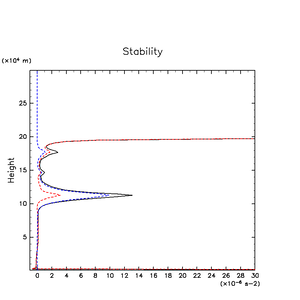### 3.4 Result (3): Static Stability

Fig. 3.5 shows the vertical profile of time and horizontal mean static stability N2 (square of buoyancy frequency N). There is a distinct maximum of N2 at the H2O condensation level. Although the peak magnitude of N2 is roughly 1/3 of that expected by the one-dimensional thermodynamic equilibrium model (See Appendix G), it can be regarded to be large enough to explain why the H2O condensation level acts as both a compositional and a dynamical boundary as shown in Section 3.2 . Fig. 3.5 also shows the contributions of the vertical gradient of mean molecular weight (mixing ratio of condensible components) and that of temperature (latent heat release) to the value of N2. The value of N2 is determined mainly by the decrease in the mean molecular weight; the contribution from temperature variation is relatively small.

The maximum values of N2 at the NH4SH reaction level and the NH3 condensation level are about 1/15 and 1/5 times as large as that at the H2O condensation level, respectively. These ratios are obviously smaller than those obtained by the one-dimensional thermodynamic equilibrium model (see Appendix G), which estimates the ratios as 1/4 and 1/3, respectively. The magnitudes of these peaks are not quite large, of the order of 10-6s-1. The reason for these weaker stabilities is that steep decreases of the mixing ratios of H2S and NH3 vapor as those observed in the one-dimensional thermodynamic equilibrium calculation do not develop around the NH3 condensation and NH4SH production levels (see Fig. 3.3). It is considered that, since the values of N2 do not grow enough, those condensation and reaction levels do not act as a compositional and/or a dynamical boundary.Figure 3.5: Vertical profiles of time and horizontal mean static stability N2 (black line). Red and blue lines indicate the contribution from temperature gradient and that from molecular weight gradient, respectively.